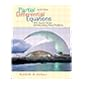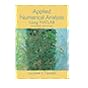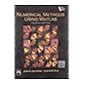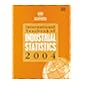# Calculus [by] Tom M. Apostol.

Material type:TextPublication details: [1967-69] Edition: 2d edDescription: 2 v. illus. 27 cmSubject(s): Mathematical analysis | CalculusDDC classification: 517
Contents:
v. 1. One-variable calculus, with an introduction to linear algebra.--v. 2. Multi-variable calculus and linear algebra, with applications to differential equations and probability.
Tags from this library: No tags from this library for this title.
Holdings
Item type Current library Call number Copy number Status Date due Barcode Item holdsBooks
Reference Stacks
517 A645c 2006 (Browse shelf(Opens below)) 01 Not For Loan 025499
Total holds: 0
##### Browsing Library, Independent University, Bangladesh (IUB) shelves, Shelving location: Reference Stacks Close shelf browser (Hides shelf browser)No cover image available515.35 N149f Fundamentals of Differential Equations and Boundary Value Problems / 515.353 A836p Partial Differential Equations With Fourier Series and Boundary Value Problems / 515.353 P887b Boundary Value Problems : and partial differential equations / 517 A645c 2006 Calculus 518 F267a Applied numerical analysis using MATLAB / 518 M4291n 2004 Numerical methods using MATLAB / 519.03 I61 International Yearbook of Industrial Statistics 2004

Includes bibliographies.

v. 1. One-variable calculus, with an introduction to linear algebra.--v. 2. Multi-variable calculus and linear algebra, with applications to differential equations and probability.

Share